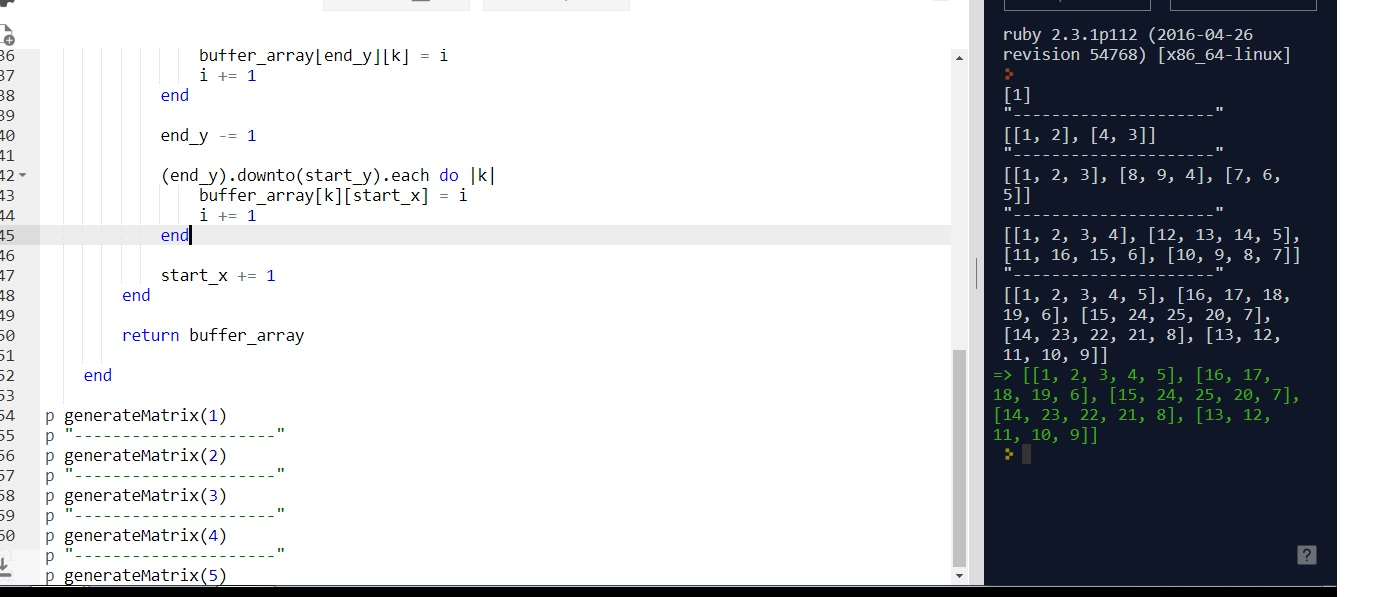# CLEAN CODE: SPIRAL ORDER MATRIX

By: Saurav

2018-01-22 10:49:00 UTC

Given an integer n, generate a square matrix filled with elements from 1 to n2 in spiral order.

Example:

Given n = 3,

You should return the following matrix:

[
[ 1, 2, 3 ],
[ 8, 9, 4 ],
[ 7, 6, 5 ]
]

InterviewBit

This problem is a pattern based problem. Its more of a how you use while and for loop rather than complex logic or algorithm.

Algo:

The spiral order filling of array elements can be done by keeping four buffer values which keep track of the start x, end x, start y and end y while filling up the
buffer array with values.

When you fill up a single dimension array inside the two square array, you need to update the variables to the next row or column.

It would be more clear walking through the code as below:

let's start with writing some tests:

```p generateMatrix(0) ==  []
p generateMatrix(1) ==  
p generateMatrix(2) == [[1, 2], [4, 3]]
p generateMatrix(3) == [[1, 2, 3], [8, 9, 4], [7, 6, 5]]
p generateMatrix(4) == [[1, 2, 3, 4], [12, 13, 14, 5], [11, 16, 15, 6], [10, 9, 8, 7]]
p generateMatrix(5) == [[1, 2, 3, 4, 5], [16, 17, 18, 19, 6], [15, 24, 25, 20, 7], [14, 23, 22, 21, 8], [13, 12, 11, 10, 9]]```

```    def generateMatrix(a)

return Array.new if a < 1
return Array.new(1,1) if a < 2

buffer_array = Array.new(a){Array.new(a, 0)}

start_x = 0
start_y = 0
end_x = a - 1
end_y = a - 1
i = 1
max = a**2

while i <= max
(start_x..end_x).each do |k|
buffer_array[start_y][k] = i
i += 1
end

start_y += 1

(start_y..end_y).each do |k|
buffer_array[k][end_x] = i
i += 1
end

end_x -= 1

(end_x).downto(start_x).each do |k|
buffer_array[end_y][k] = i
i += 1
end

end_y -= 1

(end_y).downto(start_y).each do |k|
buffer_array[k][start_x] = i
i += 1
end

start_x += 1
end

return buffer_array

end```

Works fine!Looks good to me.

### Let me know what you think! twitter: sprakash24octlinkedin

Owned & Maintained by Saurav Prakash

If you like what you see, you can help me cover server costs or buy me a cup of coffee though donation :)